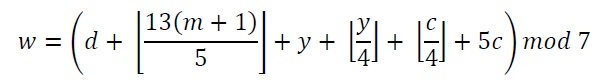# Find Weekday using Zeller's Algorithm

Zeller’s Algorithm is used to find the weekday from a given date. The formula to find weekday using Zeller’s Algorithm is here:The formula is containing some variables; They are −

d − The day of the date.

m: It is the month code. From March to December it is 3 to 12, for January it is 13, and for February it is 14. When we consider January or February, then given year will be decreased by 1.

y − Last two digits of the year

c − first two digits of the year

w − The weekday. When it is 0, it is Saturday, when it is 6, it means Friday

## Input and Output

Input:
The day, month and the year: 4, 1, 1997
Output:
It was: Saturday

## Algorithm

zellersAlgorithm(day, month, year)

Input: The date of the day.

Output: Which day it was, (Sunday to Saturday).

Begin
if month > 2, then
mon := month
else
mon := 12 + month
decrease year by 1
y := last two digit of the year
c := first two digit of the year
w := day + floor((13*(mon+1))/5) + y + floor(y/4) + floor(c/4) + 5*c
w := w mod 7
return weekday[w] //weekday will hold days from Saturday to Friday
End

## Example

#include<iostream>
#include<cmath>
using namespace std;

string weekday = {"Saturday","Sunday","Monday","Tuesday","Wednesday","Thursday","Friday"};

string zellersAlgorithm(int day, int month, int year) {
int mon;
if(month > 2)
mon = month;    //for march to december month code is same as month
else {
mon = (12+month);    //for Jan and Feb, month code will be 13 and 14
year--; //decrease year for month Jan and Feb
}

int y = year % 100;    //last two digit
int c = year / 100;    //first two digit
int w = (day + floor((13*(mon+1))/5) + y + floor(y/4) + floor(c/4) + (5*c));
w = w % 7;
return weekday[w];
}

int main() {
int day, month, year;
cout << "Enter Day: "; cin >>day;
cout << "Enter Month: "; cin >>month;
cout << "Enter Year: "; cin >>year;
cout << "It was: " <<zellersAlgorithm(day, month, year);
}

## Output

Enter Day: 04
Enter Month: 01
Enter Year: 1997
It was: Saturday